Impact Factor 2.067 | CiteScore 3.2
More on impact ›

# Frontiers in Psychology## Methods ARTICLE

Front. Psychol., 26 March 2019 | https://doi.org/10.3389/fpsyg.2019.00645

# Varimax Rotation Based on Gradient Projection Is a Feasible Alternative to SPSS

• Department of Methods and Evaluation, Institute of Psychology, University of Bonn, Bonn, Germany

## Introduction

In order to understand the approach of the present simulation study, we give a brief description of the GPR algorithm in factor and component rotation (Jennrich, 2001, 2002; Bernaards and Jennrich, 2005). More detailed descriptions of the algorithm can be found elsewhere (Mulaik, 2010). The idea of GPR is that any rotation toward simple structure relies on an optimization (minimization or maximization) criterion, where constraints are placed on some parts of the optimization function. The gradient projection algorithm can be used to solve such constraint optimization problems. A rotation of an initial m ×k loading matrix A (e.g., unrotated loadings) is given by

$Λ=AT, (1)$

where T is a k ×k transformation matrix with columns of unit length (i.e., its column sums of squares add up to 1). Let Φ be the correlation matrix between rotated factors or components. For an orthogonal rotation, the rotated components are required to remain uncorrelated, such that the k(k-1) non-diagonal elements of Φ are constrained to be zero, resulting in

$Φ=T′T=I. (2)$

The optimization criterion Q in factor and component rotation is a function of the rotated loading matrix Λ and thereby a function of T, denoted by

$f(T)=Q(Λ). (3)$

For example, the Varimax criterion seeks to maximize the variance of squared loadings (Kaiser, 1958) and is therefore a function of the transformation matrix T. The algorithm searches for a minimum of f(T), such that the Varimax criterion would be the negative of Q(Λ). The algorithm uses the negative gradient G of f(T) = Q(Λ). For orthogonal rotation, it is given by

$G=A′∂Q∂Λ. (4)$

Each rotation criterion has its own expression for ∂Q. The algorithm starts with an initial T from the manifold T that comply with the constraints. Then, T is moved by its negative gradient with step length α to find a matrix M by

$M=T−αG. (5)$

Next, M is projected back onto T by normalizing its columns to unit length. The projection is denoted by ∼T. After each step, the rotation criterion f(∼T) is evaluated and compared to the previous f(T). For a sufficiently small α, the algorithm is strictly descending, and

$f(~T)

Then, T is replaced with ∼T, and the algorithm starts again. If f (~ T) ≥ f (T), α needs to be reduced (e.g., halved), until f (~ T) < f (T). The algorithm continues until it converges, that is, when f(T) becomes minimal.

Kaiser (1958) proposes to perform a pairwise rotation of two factors or components toward the Varimax criterion. When all pairwise rotations of components are performed, the overall Varimax criterion is calculated. Then, a next cycle of rotations of all component pairs is performed, and it is checked whether the Varimax criterion has increased. This procedure is repeated until a convergence criterion is met or until a given number of cycles (i.e., a maximum number of iterations) is reached. This procedure is realized in conventional rotation software like SPSS, where the number of iterations can be specified. The number of iterations can also be specified for the GPR-Varimax algorithm, but there, the procedure is based on the gradient projection algorithm. However, the number of iterations of both rotation algorithms should not be confounded with the number of start loading matrices, which can only be specified in GPR-Varimax. Nevertheless, since the number of iterations may also affect the quality of results, we also investigate the effect of the number of iterations for GPR-Varimax and SPSS-Varimax.

Given the possibility of local optima, investigating GPR-Varimax in a simulation study requires population models that can adequately address the question of whether the algorithm finds the global optimum. Therefore, we will compare GPR-Varimax and SPSS-Varimax for two different population models. The first model is perfect orthogonal simple structure, which Varimax rotation is designed to recover (Kaiser, 1958). In the case of perfect orthogonal simple structure, there is a marked single (global) optimum for the Varimax criterion in the population. This single-optimum population model constitutes a relatively basic test for any Varimax algorithm, because local Varimax optima may only occur due to sampling error. However, if population components are weakly defined, that is, represented by few variables, the relative effect of sampling error on the loadings will be more pronounced. In this case, solutions become less stable across samples than for well-defined population components, which are represented by more variables (Guadagnoli and Velicer, 1988). Therefore, we will assess rotation performance in the single-optimum simple structure for three levels of component-definition with four, six, and eight variables per population component.

FIGURE 1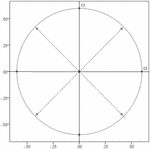Figure 1. Loadings of 24 variables on component 1 (C1) and 2 (C2) of the global optimum solution (no Kaiser normalization) given in Table 2. The dotted circle marks the size of the largest main loadings on C1 and C2, the dashed lines mark the two components of the local optimum solution.

For both the single-optimum and the double-optimum population model, rotation performance of GPR-Varimax can be evaluated using the size of the Varimax criterion itself and by assessing proximity to the global optimum of the underlying population model. If GPR-Varimax produces a substantial amount of solutions with a local optimum, the Varimax criterion should differ between solutions depending on the start loadings inserted before rotation. However, using multiple random start loading matrices and selecting the solution for which the Varimax criterion becomes maximal could result in a larger Varimax criterion as compared to using only a single start loading matrix. If GPR-Varimax generally produces solutions of a global optimum, the Varimax criterion should not substantially differ depending on the start loadings. The same pattern should be found for proximity of the sample solutions to the global optimum of the underlying population model. Proximity to population models can be computed by congruence coefficients c of the components from the solution to the respective component in the population (Tucker, 1951; Korth and Tucker, 1975). Cut-off values have been suggested to assess similarity between factors or components by congruence coefficients. For example, Lorenzo-Seva and ten Berge (2006) state that values between c = 0.85 and c = 0.94 indicate fair similarity, and that components with a congruence of c ≥ 0.95 can be regarded as equal. Mundfrom et al. (2005) use different cut-offs with c ≥ 0.98 as excellent, values between c = 0.92 and c = 0.98 as good, values between c = 0.82 and c = 0.92 as borderline, values between c = 0.68 to c = 0.82 as poor, and congruence of c ≤ 0.68 indicating terrible agreement. We will evaluate congruence coefficients of the Varimax-rotated components (SPSS and GPR) with the population components using these suggestions.

To summarize, this simulation study investigates rotation performance of GPR-Varimax in comparison to SPSS-Varimax under a number of conditions (see below). In particular, we examine whether GPR-Varimax can overcome local optima by using a sufficient number of iterations and multiple start loadings for a single-optimum and a double-optimum model of simple structure in the population.

## Materials and Methods

We used IBM SPSS Version 25 and R Version 3.5.2 to double-check results for GPR-Varimax. GPR-Varimax was performed with the syntax code for SPSS matrix and the code of the R package GPArotation. The codes can be retrieved from http://www.stat.ucla.edu/research/gpa (Bernaards and Jennrich, 2005) or copied from the Supplementary Materials of this paper. We also performed the built-in Varimax-rotation of SPSS, which is available with the SPSS command FACTOR (SPSS-Varimax).

The conditions that we manipulated refer to the population model (single-optimum and double-optimum simple structure), the number of components (k = 3, 6, 9, and 12), the number of variables per component for the single-optimum model (m/k = 4, 6, and 8), sample size (n = 100 and n = 300), the number of iterations per rotation (i = 25 and 250), and whether Varimax-rotation was based on Kaiser normalized loadings or not. Moreover, four different numbers of random start loading matrices were investigated for GPR-Varimax (q = 1, 10, 50, and 100).

### Population Factor Models

We generated two population models based on common and unique factors. The first population model was a single-optimum model of perfect orthogonal simple structure. Main loadings for the single-optimum model were a0 = 0.50 and zero on all other variables for population factors. Unique variances were ${d}_{0}=\sqrt{1-{0.50}^{2}}$ accordingly. We used m/k = 4, 6, and 8 variables per factor. Simple structure loadings of the population factors can be inspected in Table 1 for three factors and 24 variables (m/k = 6), together with the respective Varimax-rotated population components.

TABLE 1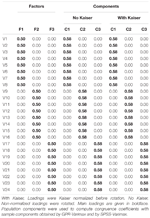Table 1. Loading matrices of the single-optimum population model for three simple structure population factors and corresponding Varimax-rotated population components.

The second population model was a double-optimum simple structure, where two competing simple structures (a stronger and a weaker one) existed in the population. We created the double-optimum structure for 2/3 of the variables and factors and kept perfect simple structure for the remaining 1/3 of the variables and factors for all conditions. For the 1/3 perfect simple structure variables and factors, main loadings remained at a0 = 0.50 and unique variances at ${d}_{0}=\sqrt{1-{0.50}^{2}}$. We defined circumplexes of two factors, where the rotational position of two factors competed against another rotational position of the two factors. For this purpose, we used 16 variables per circumplex to balance out loadings. For each circumplex, main loadings were unique for 8 variables (a1/-a1), representing the stronger simple structure, and ambiguous for the other 8 variables (a2/-a2), representing the weaker competing simple structure. Ambiguous main loadings were created by distributing the main loadings onto two factors. The loadings for three factors with ambiguous simple structure are given in Table 2. For the conditions with k = 6, 9, and 12 factors, the population loading matrices were created by means of a blockwise repetition of the loading pattern presented in Table 2.

TABLE 2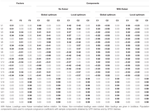Table 2. Loading matrices of the double-optimum model for three simple structure population factors and corresponding Varimax-rotated population components.

### Population Components and Data Sets

Since we investigated Varimax-rotation in PCA, we needed to obtain population components from the population factors of the models. Population components were then used to evaluate GPR-Varimax and SPSS-Varimax rotation of sample component loadings by means of congruence with the respective population components. To obtain population components, we generated population data sets on the basis of the population factor models and submitted these population data sets to PCA (see Tables 1, 2).

## Results

We compared rotation performance of GPR-Varimax and SPSS-Varimax on simulated data for the single-optimum and the double-optimum population model. We also included an analysis of real data to compare simple structure solutions from GPR-Varimax and SPSS-Varimax in an empirical setting.

### Cut-Offs for Equality of Results

TABLE 3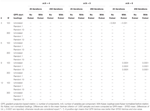Table 3. Differences in Varimax criterion between GPR-Varimax and SPSS-Varimax component rotation for single-optimum simple structure.

### Simulation Results for Single-Optimum Simple Structure

For the single-optimum population model, rotation performance of GPR-Varimax and SPSS-Varimax was extremely similar, and we found only few very slight differences Δ between them (Tables 3, 4). Values for the mean Varimax criterion in the subset of data with single-optimum simple structure ranged from v = 0.0072 (m/k = 8, k = 12, n = 100) to v = 0.0417 (m/k = 4, k = 3, n = 300). The Varimax criterion was larger for samples of n = 300 than for samples of n = 100, and Kaiser normalization of loadings before rotation yielded slightly smaller values for the Varimax criterion in small samples (n = 100). Values for mean congruence coefficients ranged from c = 0.727 (m/k = 4, k = 12, n = 100, GPR-Varimax, no Kaiser, 25 iterations per rotation) to c = 0.984 (m/k = 8, k = 3, n = 300, with and without Kaiser). Congruence increased with sample size, where all mean congruences were larger than c = 0.900 in all conditions with n = 300. The tendencies in mean Varimax criterion and congruence were found for both SPSS-Varimax and GPR-Varimax rotation.

TABLE 4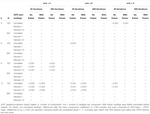Table 4. Differences in congruence coefficients with population components between GPR-Varimax and SPSS-Varimax for single-optimum simple structure.

### Simulation Results for Double-Optimum Simple Structure

For the double-optimum model with a local and a global optimum of the Varimax criterion in the population, we found more differences in rotation performance between GPR-Varimax and SPSS-Varimax than for the single-optimum population models (Tables 5, 6). The mean Varimax criterion in the subset of data with double-optimum simple structure ranged from v = 0.0066 (k = 12, n = 100, with Kaiser) to v = 0.0227 (k = 3, n = 100, no Kaiser). It decreased when loadings were Kaiser normalized before rotation. With regards to sample size, the Varimax criterion was smaller for n = 300 than for n = 100 when three or six components were rotated, but larger for n = 300 than for n = 100 for the rotation of nine and 12 components. Mean congruences were found between c = 0.725 (k = 12, n = 100, with Kaiser, SPSS-Varimax) and c = 0.936 (k = 3, n = 300, with Kaiser, GPR-Varimax with 10 random start loadings). They were overall larger in samples of n = 300 (with all c > 0.900) and when loadings were not Kaiser normalized before rotation.

TABLE 5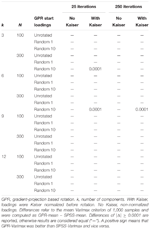Table 5. Differences in Varimax criterion between GPR-Varimax and SPSS-Varimax component rotation for double-optimum simple structure.

TABLE 6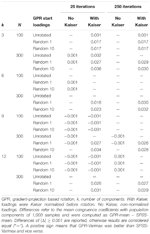Table 6. Differences in congruence coefficients with population components between GPR-Varimax and SPSS-Varimax for double-optimum simple structure.

### Empirical Example Based on a Short Knowledge Test

In addition to the simulation study, we also analyzed real data from an empirical study to show the similarity of GPR-Varimax and SPSS-Varimax in an investigative application. Therefore, PCA was performed for 17 newly developed items of a knowledge test. A total sample of 397 voluntary participants (55 females; age in years, M = 19.53, SD = 2.49) from a German school worked for about 30 min on the 17 single choice knowledge items. Each item had one correct solution and four distractors. Six items were from the knowledge domain Geography/History, five items were from the knowledge domain Science, and six items were from the knowledge domain of Culture and Arts. The item inter-correlation matrix is given in the Supplementary Material (Supplementary Table S14). Three components from the unrotated PCA explaining 30.52% of the total variance were retained for Varimax rotation (for eigenvalues of the unrotated PCA, see Table 7). Varimax rotation was performed by means of SPSS-Varimax and GPR-Varimax with a maximum of 250 iterations. As a start transformation matrix in GPR-Varimax, we used the identity matrix, one random start matrix, and 10 random start matrices. We did not use more than 10 random start matrices because the results from the simulation study yielded that using more than 10 random start matrices for the rotation of three components did not improve rotation performance.

TABLE 7

## Discussion

We assessed rotation performance of Varimax rotation in PCA based on gradient projection in comparison to the built-in SPSS-Varimax rotation in a simulation study. Referring to the discussion of multiple local optima in analytic factor and component rotation (Rozeboom, 1992; Bernaards and Jennrich, 2005; Mulaik, 2010), we examined whether the GPR-Varimax algorithm could find the global optimum of the Varimax criterion for a single-optimum and a double-optimum simple structure in the population. Therefore, we evaluated rotation performance of GPR-Varimax using unrotated loadings and multiple random start loadings. Moreover, we investigated whether selecting the best out of multiple random starts improved rotation performance of GPR-Varimax. Furthermore, we included an empirical example in which we compared Varimax solutions of GPR and SPSS for the analysis of real data.

### Summary and Interpretation of Results

Considering the results from both the single-optimum and the double-optimum population models, GPR-Varimax and SPSS-Varimax performed very similar in recovering population components. Therefore, mean congruence coefficients with population can be evaluated for the manipulated conditions for both rotation methods combined. According to the thresholds suggested by Lorenzo-Seva and ten Berge (2006), most solutions discovered by GPR-Varimax and SPSS-Varimax displayed fair similarity to population components with c > 0.85 (see Supplementary Materials). This was true for all conditions with n = 300. In the single-optimum case, all conditions with n = 300 except for k = 12 with m/k = 4 even yielded population congruence of c > 0.92, which has been suggested as threshold for good agreement by Mundfrom et al. (2005). For the other conditions, GPR-Varimax and SPSS-Varimax solutions fell into the range of borderline and poor agreement with population components, mostly depending on the number of components to be rotated, Kaiser normalization, and the population model beyond the simulated sample data sets. None of the mean congruence coefficients with population components were smaller than c = 0.68, as the threshold for terrible agreement (Mundfrom et al., 2005).

Conducting Varimax rotation by GPR and SPSS in PCA on a real data set supported the results of the simulation study. For the 17 newly developed items of a short knowledge test, both GPR-Varimax and SPSS-Varimax indicated a clear three-component solution. The recovered loading pattern was highly similar with congruence of c ≥ 0.990 between SPSS- and GPR-components, indicating excellent agreement between loading patterns (Mundfrom et al., 2005) or rather equal components (Lorenzo-Seva and ten Berge, 2006). Furthermore, the solutions did not differ between multiple start loadings in GPR-Varimax. Thus, it was not necessary to transform the unrotated start loadings from the data into random start loadings before Varimax-rotation by GPR. These findings support the notion that GPR-Varimax can be used as an alternative to SPSS-Varimax in empirical applications.

### Future Research

Future research should investigate the advantage of random starts in GPR-Varimax we found for Kaiser normalized loadings in the double-optimum case in more detail. In particular, it would be interesting to isolate the effect of random start loadings from the effect of the GPR algorithm. Therefore, one could introduce random start loadings in SPSS by writing a syntax for the SPSS MATRIX environment. These random start loadings should then be inserted in the built-in SPSS-Varimax procedure that is available by the command FACTOR to produce multiple solutions. In the same way as we proceeded with GPR-Varimax, the solutions should be successively replaced to select the one with the maximum Varimax criterion. If congruence with population components thereby reaches the level that we found for GPR-Varimax, the effect can be attributed to random starts rather than the Varimax algorithm applied. If differences between GPR-Varimax and SPSS-Varimax remain, the effect can be attributed to either the GPR-Varimax algorithm alone or a combination of multiple random starts and differences in the Varimax procedure between GPR and SPSS.

The GPR algorithm for the oblique rotation criteria Geomin and Oblimin was also used by Dien (2010), who compared different rotation methods in PCA on event-related potentials (ERP) from simulated EEG data. He found less favorable results for Geomin and Oblimin, but he could not differentiate whether the effect was due to the rotation criteria or due to the GPR-algorithm used to optimize them. One could build on these findings to examine whether GPR performs better or worse for the rotation of ERPs when compared to other rotation approaches. This should be conducted for Varimax rotation, for which misallocation of variance is a known problem in ERP data (Wood and McCarthy, 1984; Beauducel and Debener, 2003). It could be compared whether GPR-based Varimax rotation produces more or less misallocation of variance than Varimax rotation by the built-in SPSS procedure.

## Author Contributions

All authors listed have made a substantial, direct and intellectual contribution to the work, and approved it for publication.

## Conflict of Interest Statement

The authors declare that the research was conducted in the absence of any commercial or financial relationships that could be construed as a potential conflict of interest.

## Supplementary Material

The Supplementary Material for this article can be found online at: https://www.frontiersin.org/articles/10.3389/fpsyg.2019.00645/full#supplementary-material

## References

Beauducel, A., and Debener, S. (2003). Misallocation of variance in event-related potentials: simulation studies on the effects of test power, topography, and baseline-to-peak versus principal component quantifications. J. Neurosci. Methods 124, 103–112. doi: 10.1016/S0165-0270(02)00381-3

Bernaards, C. A., and Jennrich, R. I. (2005). Gradient projection algorithms and software for arbitrary rotation criteria in factor analysis. Educ. Psychol. Meas. 65, 676–696. doi: 10.1177/0013164404272507

Browne, M. W. (2001). An overview of analytic rotation in exploratory factor analy-sis. Multivariate Behav. Res. 36, 111–150. doi: 10.1207/S15327906MBR3601_05

Carroll, J. B. (1953). An analytical solution for approximating simple structure in factor analysis. Psychometrika 18, 23–38. doi: 10.1007/BF02289025

Costa, P. T., and McCrae, R. R. (2006). Revised NEO Personality Inventory (NEO PI-R): Manual (UK Edition). Oxford: Hogrefe.

Dien, J. (2010). Evaluating two-step PCA of ERP data with Geomin, Infomax, Oblimin, Promax, and Varimax rotations. Psychophysiology 47, 170–183. doi: 10.1111/j.1469-8986.2009.00885.x

Eysenck, H. J. (1991). Dimensions of personality: 16, 5 or 3?—Criteria for a taxonomic paradigm. Personal. Individ. Differ. 12, 773–790. doi: 10.1016/0191-8869(91)90144-Z

Eysenck, H. J., Barrett, P., Wilson, G., and Jackson, C. (1992). Primary trait measurement of the 21 components of the P-E-N system. Eur. J. Psychol. Assess. 8, 109–117.

Fabrigar, L. R., Wegener, D. T., MacCallum, R. C., and Strahan, E. J. (1999). Evaluating the use of exploratory factor analysis in psychological research. Psychol. Methods 4, 272–299. doi: 10.1037//1082-989X.4.3.272

Gorsuch, R. L. (1983). Factor Analysis, 2nd Edn. Hillsdale, NJ: Erlbaum.

Grice, J. W. (2001). A comparison of factor scores under conditions of factor obliquity. Psychol. Methods 6, 67–83. doi: 10.1037//1082-989X.6.1.67

Guadagnoli, E., and Velicer, F. W. (1988). Relation of sample size to the stability of component patterns. Psychol. Bull. 103, 265–275. doi: 10.1037/0033-2909.103.2.265

Gurtman, M. B. (1992). Construct validity of interpersonal personality measures: the interpersonal circumplex as a nomological net. J. Personal. Soc. Psychol. 63, 105–118. doi: 10.1037/0022-3514.52.5.1019

Harman, H. H. (1967). Modern Factor Analysis, 2nd Edn. Chicago: University of Chicago Press.

Hattori, M., Zhang, G., and Preacher, K. J. (2017). Multiple local solutions and Geomin rotation. Multivariate Behav. Res. 52, 720–731. doi: 10.1080/00273171.2017.1361312

Hendrickson, A. E., and White, P. O. (1964). Promax: a quick method for rotation to oblique simple structure. Br. J. Stat. Psychol. 17, 65–70. doi: 10.1111/j.2044-8317.1964.tb00244.x

Hofstee, W. K., de Raad, B., and Goldberg, L. R. (1992). Integration of the Big Five and circumplex approaches to trait structure. J. Personal. Soc. Psychol. 63, 146–163. doi: 10.1037//0022-3514.63.1.146

Jennrich, R. I. (2001). A simple general procedure for orthogonal rotation. Psychometrika 66, 289–306. doi: 10.1007/BF02294840

Jennrich, R. I. (2002). A simple general method for oblique rotation. Psychometrika 67, 7–19. doi: 10.1007/BF02294706

Kaiser, H. F. (1958). The varimax criterion for analytic rotation in factor analysis. Psychometrika 23, 187–200. doi: 10.1007/BF02289233

Kiers, H. A. L. (1994). Simplimax: oblique rotation to an optimal target with simple structure. Psychometrika 59, 567–579. doi: 10.1007/BF02294392

Korth, B., and Tucker, L. R. (1975). The distribution of chance congruence coefficients from simulated data. Psychometrika 40, 361–372. doi: 10.1007/BF02291763

Lee, K., and Ashton, M. C. (2004). Psychometric properties of the HEXACO personality inventory. Multivariate Behav. Res. 39, 329–358. doi: 10.1207/s15327906mbr3902_8

Lorenzo-Seva, U., and Ferrando, P. J. (2006). FACTOR: a computer program to fit the exploratory factor analysis model. Behav. Res. Methods 38, 88–91. doi: 10.3758/BF03192753

Lorenzo-Seva, U., and ten Berge, J. F. M. (2006). Tucker’s congruence coefficient as a meaningful index of factor similarity. Methodology 2, 57–64. doi: 10.1027/1614-2241.2.2.57

MacCallum, R. C., Widaman, K. F., Zhang, S., and Hong, S. (1999). Sample size in factor analysis. Psychol. Methods 4, 84–99. doi: 10.1037/1082-989X.4.1.84

Mulaik, S. A. (2010). Foundations of Factor Analysis, 2nd Edn. Boca Raton, FL: Chapman & Hall.

Mundfrom, D. J., Shaw, D. G., and Ke, T. L. (2005). Minimum sample size recommendations for conducting factor analyses. Int. J. Test. 5, 159–168. doi: 10.1207/s15327574ijt0502_4

Muthén, L. K., and Muthén, B. O. (2015). MPLUS. Los Angeles, CA: Muthén & Muthén.

Rozeboom, W. W. (1992). The glory of suboptimal factor rotation: why local minima in analytic optimization of simple structure are more blessing than curse. Multivariate Behav. Res. 27, 585–599. doi: 10.1207/s15327906mbr2704_5

Trendafilov, N. T., and Jolliffe, I. T. (2006). Projected gradient approach to the numerical solution of the SCoTLASS. Comput. Stat. Data Anal. 50, 242–253. doi: 10.1016/j.csda.2004.07.017

Tucker, L. R. (1951). A Method for Synthesis of Factor Analysis Studies. Personnel Research Section Report, No.984. Washington, DC: Department of the Army. doi: 10.21236/AD0047524

Wood, C., and McCarthy, G. (1984). Principal component analysis of event-related potentials: simulation studies demonstrate misallocation of variance across components. Electroencephalogr. Clin. Neurophysiol. 59, 249–260. doi: 10.1016/0168-5597(84)90064-9

Citation: Weide AC and Beauducel A (2019) Varimax Rotation Based on Gradient Projection Is a Feasible Alternative to SPSS. Front. Psychol. 10:645. doi: 10.3389/fpsyg.2019.00645

Received: 14 September 2018; Accepted: 07 March 2019;
Published: 26 March 2019.

Edited by:

Holmes Finch, Ball State University, United States

Reviewed by:

Paul T. Barrett, Independent Researcher, Auckland, New Zealand
Guangjian Zhang, University of Notre Dame, United States